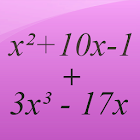Polynomial Calculator

All Android applications categories

All Android games categories# Polynomial Calculator

by: 934 6.4

6.4 Users
rating

## Screenshots

Description

This app is a free math calculator which allows you to calculate with polynomials. Yor are able to multiply, divide, add and subtract polynomials.

Best mathematical tool for school and college! If you are a student, it will help you to learn algebra!

Note: Polynomials appear in settings ranging from basic chemistry and physics to economics and social science; they are used in calculus and numerical analysis to approximate other functions. In advanced mathematics, polynomials are used to construct polynomial rings, a central concept in algebra and algebraic geometry.

Tags: polynom rechner , polynome multiplizieren rechner , polynomial calculator , polynomial to binary calculator , calculadora polinomica , polynomdarstellung rechner , polynomial calculator binary , vyjujxktysrfkmrekznjh , app raiz cuadrada de ecuaciones polinomicas

from 934 reviews

"Good"

6.4